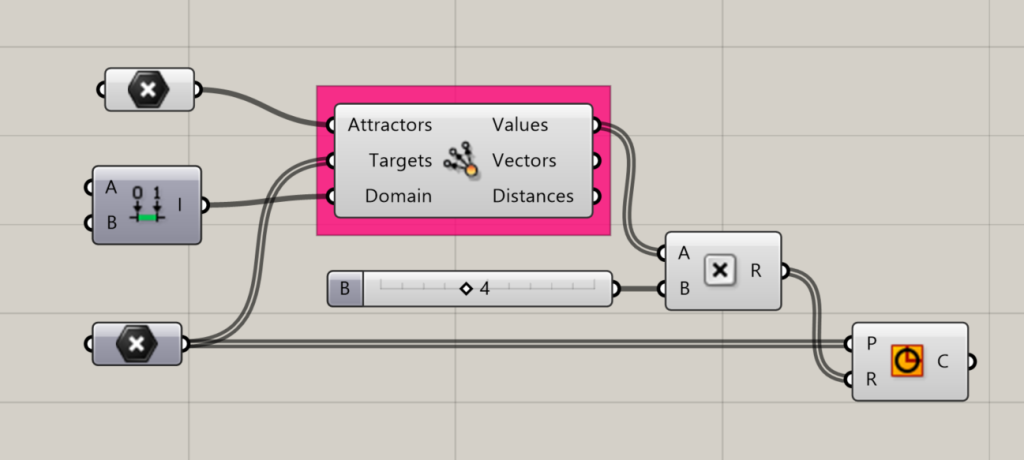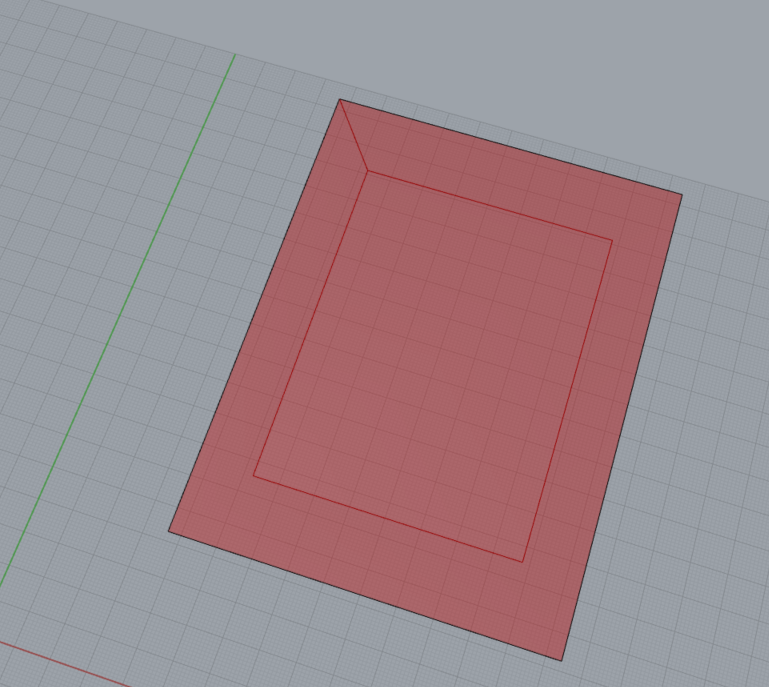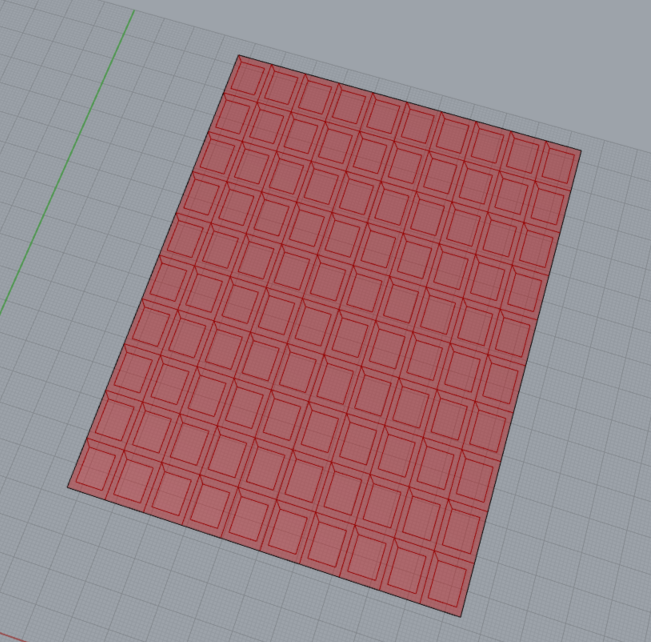# Generate

The Generate components allow users to subdivide panels recursively and create attractor/target relationships.

### Appearance

#### Attractor

Measures the distance between an Attractor point or list of points and a series of Target points, and generates values within the given domain relative to the distance. Also returns vectors and a list of distances.

Input:

• A list of points for the Attractors.
• A list of points to become Targets.
• A domain to apply to the values.

Returns:

• The component assigns Values within the specified domain related to the distance between the Attractor point and each Target point.
• A list of vectors between the Attractor point and Target point
• A numerical list of the Distances between the Attractor point and the Target PointsAttractor example, where the size of a series of circles is being determined by their distance from a designated Attractor point.

#### Attractor Wave

Measures the distance between an Attractor point or list of points and a series of Target points, and generates values within the given domain relative to the distance, applying a wave function that creates variation in the results. Also returns vectors and a list of distances.

Input:

• A list of points for the Attractors.
• A list of points to become Targets.
• A domain to apply to the values.
• A frequency for the wave form.

Returns:

• A list of the resulting values.
• A list of vectors between the Attractor point and Target point
• A numerical list of the Distances between the Attractor point and the Target Points

Divides a surface into nested series of three equally sized subdivisions.

Input:

• A list of triangular surfaces. This component was designed to work with Lunchbox’s triangular paneling components.
• The number of times you wish to subdivide the surface into 3 equally sized quadrangles.
• A boolean toggle to set the Recursion type. There are two options available (resulting in “0” and “1” as index numbers), so a boolean toggle is an effective way to toggle between the two styles.
• A boolean toggle to indicate if the results should be created as wireframes or surfaces. To create surfaces, set to false. To create wires, set to true.

Returns: A series of subdivided surfaces.

Tip: Do not set the subdivisions to a high number in your initial setup. In the example to the right, you can see that it exponentially divides!

#### Panel Frame

Creates an offset frame using a panel.

Input:

• A list of panels as planar surfaces
• A scale factor which will set the size of the resulting panel as a proportion of the whole.
• Use a factor <1 to inset the panel
• Use a factor >1 to offset the panel

Returns: A list of Frame surfaces and a List of panel surfaces.Single panel example, where the frame is inset by a scale factor of 0.7. The resulting panel is 70% of the size of the input surface.The component was designed to work with Lunchbox’s paneling tools. This example creates frames for each panel created on a surface with the QuadPanel component.

Input:

• A list of quadrangular panels
• The desired number of divisions
• Boolean toggles for the inputs labeled B1-B4. Determines whether a subdivided panel should be subdivided beyond the initial subD.
• A boolean toggle to indicate if the results should be created as wireframes or surfaces. To create surfaces, set to false. To create wires, set to true.

Returns:

• Two lists of subdivided Quadrangles (either surfaces or curves depending on the Wire setting).

#### Subdivide Triangle

Description text

Input:

• A list of triangular panels
• The desired number of divisions
• Boolean toggles for the inputs labeled B1-B4. Determines whether a the panel should be subdivided beyond the initial subD.
• A boolean toggle to indicate if the results should be created as wireframes or surfaces. To create surfaces, set to false. To create wires, set to true.

Returns:

• Two lists of subdivided Triangles (either surfaces or curves depending on Wire setting).